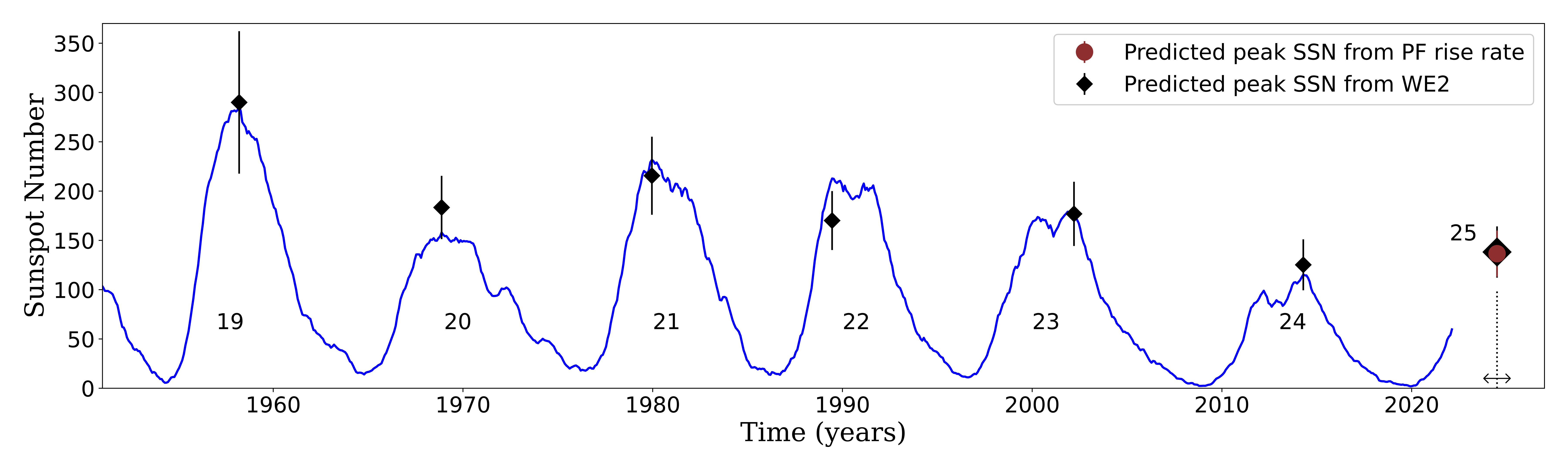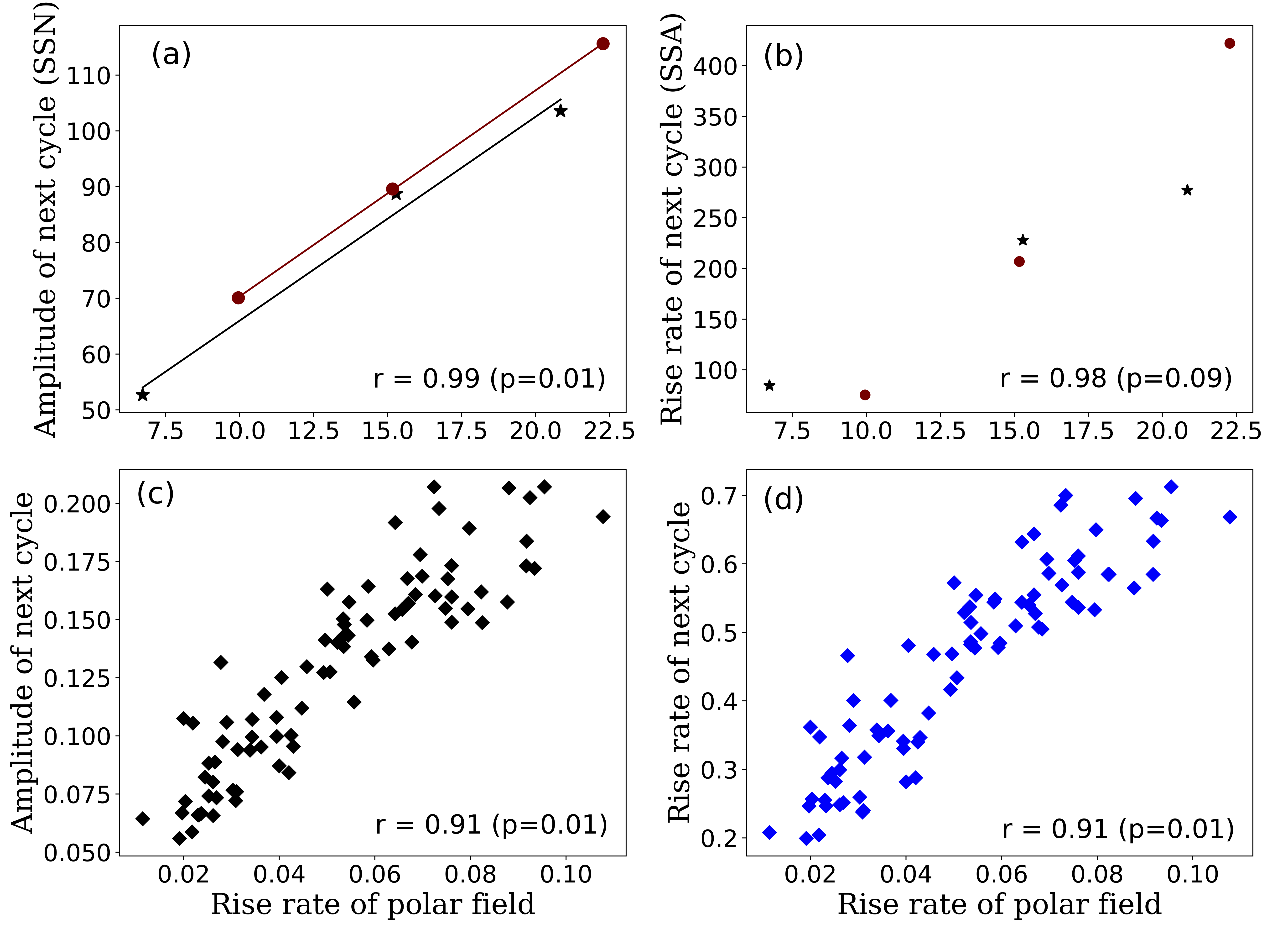# 178. Prediction based on the polar field rise rate suggests that Solar Cycle 25 will be slightly stronger than Cycle 24

Contributed by Bidya Karak. Posted on April 28, 2022

Pawan Kumar, Akash Biswas, Bidya Binay Karak
Department of Physics, Indian Institute of Technology (Banaras Hindu University), Varanasi 221005, India

The number of sunspots that appears on the solar surface varies in a cyclic fashion with a cycle period of about 11 years. This is famously known as the sunspot cycle. The strength of the solar magnetic field is strongly coupled with the activity level of the sunspot cycle. It is the dynamic nature of the solar magnetic fields that give rise to highly energetic transient events like CMEs or solar flares which impact the space weather and earth severely. In our highly space-based technology-dependent civilization, securing the space-based assets from the adverse effects of the space weather is of utmost importance. The early prediction of the strength of an upcoming solar cycle can give us a significant advantage in this aspect. In our previous article, we showed that the polar field, 4 years after the polarity reversal, can be used to make a reasonable prediction of the amplitude of the next solar cycle. In our recent Letter, we presented a novel technique for predicting the strength of an upcoming cycle. It also broadens the temporal scope of our early prediction to a significant extent.

An important feature of the sunspot cycle is that stronger cycles rise faster than weaker ones.
This feature, called WE2, is an aspect of the Waldmeier effect. This feature enables us to establish a linear relationship between the rise rate and the amplitude of the cycle. From the observational data of last 13 cycles, we got a linear correlation coefficient of 0.87 between these two quantities. Now, as the current cycle 25 has just undergone around the first 2 years of its rise, we can calculate the rise rate of the cycle from this data, and using the linear regression relation, we calculate the amplitude of the sunspot Cycle 25 to be 138 ± 26 and the cycle will reach its peak around the mid- to late-2024. To test the reliability of this method, we predict the amplitudes of the last few observed cycles and as it can be seen from Figure 1, we get most of the predicted values to be in agreement with the observations.Figure 1| Comparison of our prediction with observations. Temporal variation of the observed SSN is shown by the blue curve. Squares and vertical lines represent the predicted amplitudes and their errors based on the rise rate (WE2). The time of the peak of predicted Cycle 25 is shown by the vertical dotted line with the error by a horizontal arrow. The prediction for Cycle 25 using the rise rate of the previous cycle’s polar field is shown by a (dark red) filled circle.

To predict the solar cycle at an earlier phase of its evolution, the polar precursor method is implemented in which, the amplitude of the polar field at a cycle minimum is taken as the seed to predict the amplitude of the next cycle. By analyzing the data of the amplitude of the polar field at the previous minimum and the rise rate of a cycle, we get a very strong correlation between these two quantities both in observation and dynamo models. This correlation indicates that a strong poloidal field at a cycle indeed makes the following cycle rise faster and it becomes stronger obeying WE2.

Finally, further going one step backward in the evolution of the solar cycles, we find a very interesting feature, the rise rate of the polar field build-up after the polarity reversal is strongly correlated with both the rise rate and the amplitude of the next cycle; see Figure 2. This result indeed implies that there is a physical link between the polar field build-up and the rise rate of the next cycle. And WE2 enables us to predict the amplitude of a cycle from the rise rate of the polar field calculated from the data of a few years after the polarity reversal of the previous cycle. By computing the rise rate of the polar field during the first 3 years of the decline phase of Cycle 24 and using the regression relation shown in Figure 2(a), we predict that the peak of cycle 25 will be around 137 ± 23. This value is very close to the one we got directly using WE2.Figure 2| Scatter plots between the rise rate of polar field with next cycle amplitude and rise rate. (a) and (b) are obtained from observational data (asterisks and filled circles are for the northern and southern hemispheres respectively), while (c) and (d) are from the dynamo model.

This work shows a strong physical connection between the polar field build-up rate and the next cycle amplitude. Furthermore, it also shows that the Waldmeier effect is physically related with this build-up rate of the polar field. Our prediction based on the WE2 is 138 ± 26, while based on the polar field rise rate is 137±23. Quite close predictions in these methods suggest that a reliable prediction of the amplitude of a cycle can be made as early as about 9 years before it reaches its peak; see Ref.  for details.

### References

 Kumar P., Nagy M., Lamerle M., Karak B. B., Petrovay K., 2021, ApJ, 909, 87
 Kumar P.,Biswas A.,Karak B. B., ref: https://ui.adsabs.harvard.edu/abs/2022arXiv220311494K
 Karak B. B., Choudhuri A. R., 2011, MNRAS, 410, 1503
 Schatten K. H., Scherrer P. H., Svalgaard L., Wilcox J. M., 1978, Geophys. Res. Lett., 5, 411